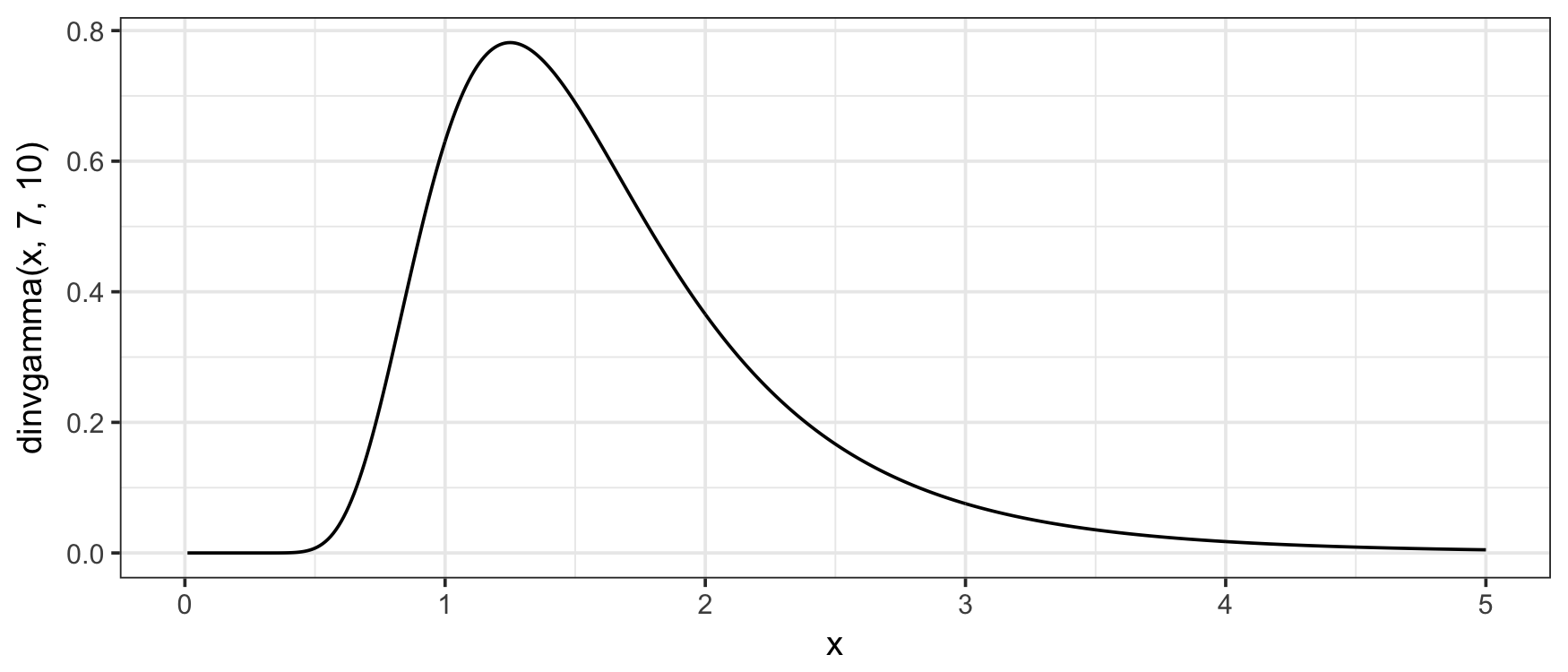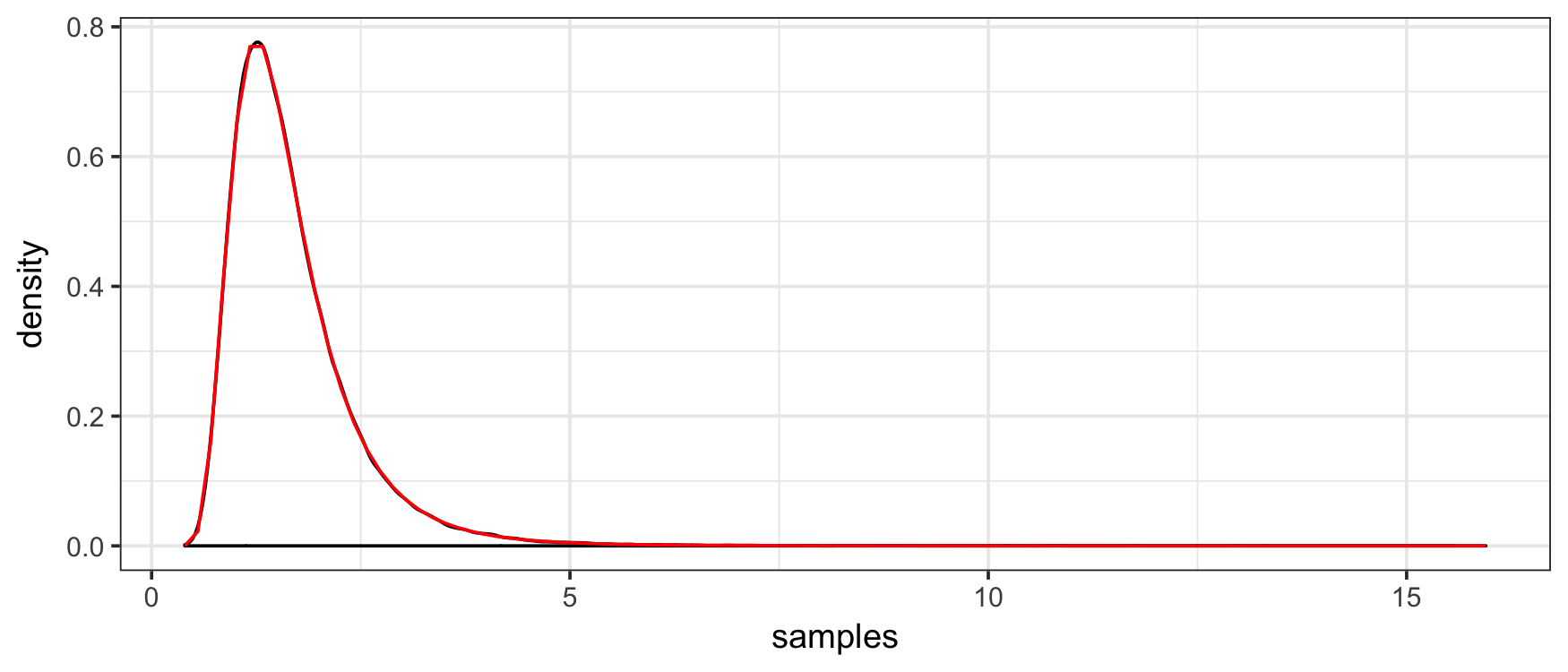# invgamma

invgamma implements the `(d/p/q/r)` statistics functions for the inverse gamma distribution in R. It is ideal for using in other packages since it is lightweight and leverages the `(d/p/q/r)gamma()` line of functions maintained by CRAN.

### Getting invgamma

There are two ways to get invgamma. For the CRAN version, use

``install.packages("invgamma")``

For the development version, use

``````# install.packages("devtools")
devtools::install_github("dkahle/invgamma")``````

### The `(d/p/q/r)invgamma()` functions

The functions in invgamma match those for the gamma distribution provided by the stats package. Namely, it uses as its density f(x) = (b^a / Gamma(a)) x^-(a+1) e^(-b/x), where a = `shape` and b = `rate`.

The PDF (the f(x) above) can be evaluated with the `dinvgamma()` function:

``````library(invgamma)
library(ggplot2); theme_set(theme_bw())
x <- seq(0, 5, .01)
qplot(x, dinvgamma(x, 7, 10), geom = "line")
#  Warning: Removed 1 rows containing missing values (geom_path).``````The CDF can be evaluated with the `pinvgamma()` function:

``````f <- function(x) dinvgamma(x, 7, 10)
q <- 2
integrate(f, 0, q)
#  0.7621835 with absolute error < 7.3e-05
(p <- pinvgamma(q, 7, 10))
#   0.7621835``````

The quantile function can be evaluated with `qinvgamma()`:

``````qinvgamma(p, 7, 10) # = q
#   2``````

And random number generation can be performed with `rinvgamma()`:

``````set.seed(1)
rinvgamma(5, 7, 10)
#   1.9996157 0.9678268 0.9853343 1.3157697 3.1578177``````

`rinvgamma()` can be used to obtain a Monte Carlo estimate of the probability given by `pinvgamma()` above:

``````samples <- rinvgamma(1e5, 7, 10)
mean(samples <= q)
#   0.7621``````

Moreover, we can check the consistency and correctness of the implementation with

``````qplot(samples, geom = "density") +
stat_function(fun = f,  color = "red")``````### The `(d/p/q/r)invchisq()` and `(d/p/q/r)invexp()` functions

The gamma distribution subsumes the chi-squared and exponential distributions, so it makes sense to include the `*invchisq()` and `*invexp()` functions in invgamma. Their implementations, however, wrap `*chisq()` and `*exp()`, not `*invgamma()`.